UPSC  >  NCERT Solutions: Chemical Reactions & Equations

# Chemical Reactions & Equations NCERT Solutions - Science & Technology for UPSC CSE

PAGE NO. 6

Q.1. Why should a magnesium ribbon be cleaned before burning in air?
Ans. When
magnesium ribbon is exposed to air, it forms a layer of magnesium oxide on its surface. This layer of magnesium oxide, being a stable compound, prevents further reaction of magnesium with oxygen. Hence, it should be cleaned before burning in air to remove this layer so that the metal can be exposed to air properly (the oxide layer may prevent or slow down the burning of magnesium.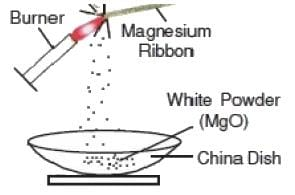Fig: Burning of magnesium ribbon

Q.2. Write the balanced equation for the following chemical reactions.
(a) Hydrogen + Chlorine → Hydrogen chloride
(b) Barium chloride + Aluminium sulphate → Barium sulphate + Aluminium chloride
(c) Sodium + Water → Sodium hydroxide + Hydrogen
Ans.

(a) H2(g) + Cl2(g) → 2HCl(g)
(b) 3BaCl2 (s) + Al2(SO4)3(s) → 3BaSO4(s) + 2AlCl3(s)
(c) 2Na(s) + 2H2O(l) → 2NaOH(aq) + H2(g)

Q.3. Write a balanced chemical equation with state symbols for the following reactions.
(a) Solutions of barium chloride and sodium sulphate in water react to give insoluble barium sulphate and the solution of sodium chloride.
(b) Sodium hydroxide solution (in water) reacts with a hydrochloric acid solution (in water) to produce sodium chloride solution and water.

Ans.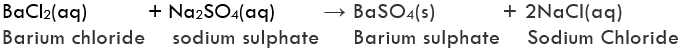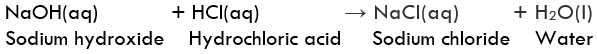PAGE NO. 10

Q.1. A solution of a substance ‘X’ is used for whitewashing.
(a) Name the substance ‘X’ and write its formula.
(b) Write the reaction of the substance ‘X’ named in (i) above with water.

Ans.
(a) The substance ‘X’ is calcium oxide. Its chemical formula is CaO.
(b) Calcium oxide reacts vigorously with water to form calcium hydroxide (slaked lime).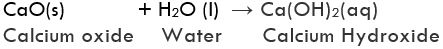Q.2. Why is the amount of gas collected in one of the test tubes in Activity 1.7 double the amount collected in the other? Name this gas.
Ans.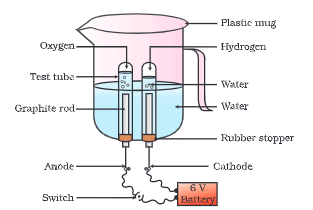Fig: Electrolysis of Water

In Activity 1.7, gas collected in one of the test tubes is double of the amount collected in the other. This can be explained with the help of the chemical reaction that takes place.

2H2O (l)→ 2H2(g) + O2(g)

The volume of the gas is proportional to the number of molecules of the gas as indicated in the balanced equation. There are two molecules of hydrogen and one molecule of oxygen in the balanced equation. Hence the amount of Hydrogen collected would be double than that of oxygen.

PAGE NO. 13

Q.1. Why does the colour of copper sulphate solution change when an iron nail is dipped in it?
Ans. Iron is more reactive than copper. So, When an iron nail is dipped in a copper sulphate solution, iron displaces copper from its solution to form iron sulphate, which is green in colour.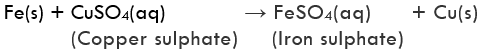Hence, the blue colour of copper sulphate solution changes into green colour because of this displacement reaction.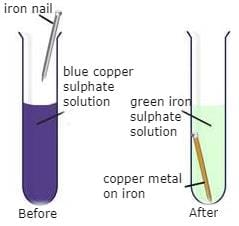Fig: Iron nail dipped in copper sulphate solution

Q.2. Give an example of a double displacement reaction other than the one given in Activity 1.10.
Ans. Sodium carbonate and calcium chloride exchange ions to form two new compounds calcium carbonate and sodium chloride.
Na2CO3(aq) + CaCl2(aq) CaCO3(s) + 2NaCl(aq)

Q.3. Identify the substances that are oxidised and the substances that are reduced in the following reactions.
(a) 4Na (s)+ O2 (g) → 2Na2O (s)
(b) CuO (s) +H2 (g) → Cu (s)+ H2O (l)

Ans.
(a) Sodium (Na) is oxidised as it gains oxygen and oxygen gets reduced.
(b)
Copper oxide (CuO) is reduced to copper (Cu) while hydrogen (H2) gets oxidised to water (H2O).

PAGE NO. 14 - 16

EXERCISE QUESTIONS
Q.1. Which of the statements about the reaction below are incorrect?
2PbO (s) + C (s) → 2Pb (s) + CO2 (g)
(b) Carbon dioxide is getting oxidised.
(c) Carbon is getting oxidised.
(d) Lead oxide is getting reduced.

(i) (a) and (b)
(ii) (a) and (c)
(iii) (a), (b) and (c)
(iv) all

Ans.
(b) Carbon dioxide is getting oxidised is an incorrect answer.
(c) Carbon is getting oxidised is an correct answer because the removed oxygen from lead is added to the elemental Carbon.
(d) Lead oxide is getting reduced is correct answer because oxygen is being removed.
As statements (a) and (b) are incorrect, answer (i) is correct.

Q.2. Fe2O3 + 2Al → Al2O + 2Fe
The above reaction is an example of a
(a) combination reaction.
(b) double displacement reaction.
(c) decomposition reaction.
(d) displacement reaction.

Ans. (d)
Solution.
In this reaction Aluminum is more reactive metal than Fe. The Oxygen from the Ferrous oxide is getting displaced to the Aluminium metal to form Aluminium Oxide. Therefore Al will displace Fe from its oxide. Since one-time displacement is occurring, therefore, it is called a single displacement reaction.

Q.3. What happens when dilute hydrochloric acid is added to iron filings? Tick the correct answer.
(a) Hydrogen gas and iron chloride are produced.
(b) Chlorine gas and iron hydroxide are produced.
(c) No reaction takes place.
(d) Iron salt and water are produced.

Ans. (a)
Solution.
Fe(s) + 2HCl(dil.) → FeCl2(aq) + H2(g)
Hydrogen gas and Iron (II) chloride are produced.

Q.4. What is a balanced chemical equation? Why should chemical equations be balanced?

Ans. Balanced chemical equation means total number of atoms of each element should be equal on both sides of the reaction.
For example, magnesium and oxygen combine, when heated to form a single compound magnesium oxide.
2Mg(s) + O2(g) → 2MgO(s)
The reaction should be balanced because matter can neither be created nor be destroyed. The total mass of reactants should be equal to the total mass of products.

Q.5. Translate the following statements into chemical equations and then balance them.
(a) Hydrogen gas combines with nitrogen to form ammonia.
(b) Hydrogen sulphide gas burns in air to give water and sulphur dioxide.
(c) Barium chloride reacts with aluminium sulphate to give aluminium chloride and a precipitate of barium sulphate.

(d) Potassium metal reacts with water to give potassium hydroxide and hydrogen gas.
Ans.
(a) Unbalanced equation: H2(g) + N2(g) NH3(g)
Balanced equation: 3H2(g) + N2(g) → 2NH3(g)
(b) Unbalanced equation: H2S + O→ H2O + SO2
Balanced equation: 2H2S (g) + 3O2(g) → 2H2O(l) + 2SO2(g)
(c) Unbalanced equation: BaCl2(aq) + Al2(SO4)3(aq)  → AlCl3(aq) + BaSO4(s)
Balanced equation: 3BaCl2(aq) + Al2(SO4)3(aq) → 2AlCl3(aq) + 3BaSO4(s)
(d) Unbalanced equation: K(s) + H2O(l) → KOH (aq) + H2(g)
Balanced equation: 2K(s) + 2H2O(l) → 2KOH(aq) + H2(g)

Q.6. Balance the following chemical equations.
(a) HNO3 + Ca(OH)2 → Ca(NO3)2 + H2O
(b) NaOH + H2SO4 → Na2SO4 + H2O
(c) NaCl + AgNO3 → AgCl + NaNO3
(d) BaCl2 + H2SO4 → BaSO4 + HCl

Ans. Balanced chemical equations are given as under :

(a) 2HNO3(aq) + Ca(OH)2(aq) Ca(NO3)2(aq) + 2H2O(l)
(b) 2NaOH(aq) + H2SO4(aq) → Na2SO4(aq) + 2H2O(l)
(c) NaCl(aq) + AgNO3(aq) → AgCl(s) + NaNO3(aq)
(d) BaCl2(aq) + H2SO4(aq) → BaSO4(s) + 2HCl(aq)

Q.7. Write the balanced chemical equations for the following reactions.
(a) Calcium hydroxide + Carbon dioxide → Calcium carbonate + Water
(b) Zinc + Silver nitrate → Zinc nitrate + Silver
(c) Aluminium + Copper chloride → Aluminium chloride + Copper
(d) Barium chloride + Potassium sulphate → Barium sulphate  + Potassium chloride

Ans. Balanced chemical equations for the reactions are given as under:

(a) Ca(OH)2(aq) + CO2(g) → CaCO3(s) + H2O(l)
(b) Zn(s) + 2AgNO3(aq) → Zn(NO3)2(aq) + 2Ag(s)
(c) 2Al(s) + 3CuCl2(aq) → 2AlCl3(aq) + 3Cu(s)
(d) BaCl2(aq) + K2SO4(aq) → BaSO4(s) + 2KCl(aq)

Q.8. Write the balanced chemical equation for the following and identify the type of reaction in each case.
(a)Potassium bromide (aq) + Barium iodide (aq)­ → Potassium iodide (aq) + Barium bromide(s)
(b) Zinc carbonate (s) → Zinc oxide (s)  + Carbon dioxide (g)
(c) Hydrogen (g) + Chlorine (g) → Hydrogen chloride (g)
(d) Magnesium (s) + Hydrochloric acid (aq) → Magnesium chloride(aq) + Hydrogen (g)

Ans.

(a) 2KBr(aq) + BaI2(aq) → 2KI(aq) + BaBr2(s)
Double displacement reaction
(b) ZnCO3(s) →  ZnO(s) + CO2(g)
Decomposition reaction
(c) H2(g) + Cl2(g) → 2HCl(g)
Combination reaction
(d) Mg(s) + 2HCl(aq) → MgCl2(aq) + H2(g)
Displacement Reaction

Q.9. What does one mean by exothermic and endothermic reactions? Give examples.
Ans. Endothermic Reaction: An endothermic reaction occurs when energy is absorbed from the surroundings in the form of heat.

Examples: Photosynthesis, melting of ice, evaporation.
Exothermic Reaction: Conversely, an exothermic reaction is one in which energy is released from the system into the surroundings.
Examples: Explosions, concrete setting, nuclear fission and fusion.

Q.10. Why is respiration considered an exothermic reaction? Explain.
Ans. During respiration, glucose combines with oxygen in the cells of our body and provides energy. As energy is released during respiration, therefore, respiration is regarded as exothermic reaction.
C6H12O(aq) + 6O2(g) → 6CO2(g) + 6H2O(l) + Energy

Q.11.
Why are decomposition reactions called the opposite of combination reactions? Write equations for these reactions.
Ans. Decomposition reactions are those in which a compound breaks down to form two or more substances. These reactions require a source of energy to proceed. Thus, they are the exact opposite of combination reactions in which two or more substances combine to give a new substance with the release of energy. For Example of decomposition Reactions are:

ZnCO3 → ZnO + CO2
CaCO3 + Energy → CaO + CO2
2HgO → 2Hg + O2

Q.12. Write one equation each for decomposition reactions where energy is supplied in the form of heat, light or electricity.
Ans.
(a) Thermal decomposition reaction (Thermolysis): When decomposition is carried out by heating, it is called thermal decomposition. On heating, Calcium carbonate decomposes to calcium oxide and carbon dioxide as shown below:
CaCO3(s) → CaO(s) + CO2(g)
(b) Photodecomposition reaction (Photolysis): When decomposition is carried out by light, it is called photolysis. The below reaction is used in black and white photography.
2AgCl(s)   2Ag(s)  + Cl2 (g)
(c) Electrolytic decomposition reaction (Electrolysis): When decomposition is carried out by electricity, it is called electrolysis. On passing electricity, water decomposes to hydrogen and oxygen.
2H2O (l) 2H2(g) + O2(g)

Q.13. What is the difference between displacement and double displacement reactions? Write equations for these reactions.
Ans. Displacement reaction: Those reactions in which more reactive metal displaces less reactive metal from its salt solution is called displacement reactions.
Fe(s) + CuSO4(aq) → FeSO4(aq) + Cu(s)
Double displacement reaction: Those reactions in which two compounds exchange their ions to form two new compounds are called double displacement reactions.
HCl(aq) + NaOH(aq) → NaCl(aq) + H2O(l)

Q.14. In the refining of silver, the recovery of silver from silver nitrate solution involved displacement by copper metal. Write down the reaction involved.
Ans.
Cu(s) + 2AgNO3(aq) → Cu(NO3)2(aq) + 2Ag(s)

Q.15. What do you mean by a precipitation reaction? Explain by giving examples.
Ans.
When two solutions containing soluble salts are combined, a double displacement reaction takes place in which the ions are exchanged between the compounds. When one of such compounds is formed  in solid form (that is insoluble in aqua) then it settles down at the bottom of the container. This solid is known as the precipitate and the respective reaction is termed as the precipitation reaction. Few examples of precipitation reactions are:
When solutions of silver nitrate and sodium chloride are mixed, white precipitate of silver chloride is formed.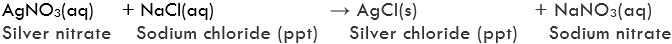On adding dilute hydrochloric acid to the aqueous solution of lead nitrate, precipitate of lead chloride is formed.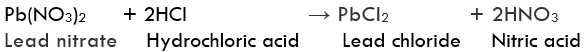Q.16. Explain the following in terms of gain or loss of oxygen with two examples each.
(a) Oxidation
(b) Reduction

Ans. (a) Oxidation: It is a process in which gain of oxygen takes place

Examples: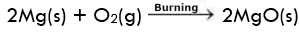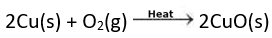(b) Reduction: It is a process in which removal of oxygen takes place.
Examples: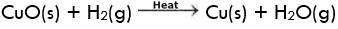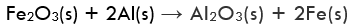Q.17. A shiny brown-coloured element ‘X’ on heating in air becomes black in colour. Name the element ‘X’ and the black coloured compound formed.
Ans. ‘X’ is Copper.

Copper gets oxidised to copper oxide which is black in colour.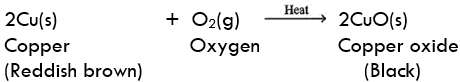Q.18. Why do we apply paint on iron articles?
Ans. Painting is done so as to prevent iron from rusting. When the surface of iron is coated with paint, its surface does not come in contact with oxygen and moisture and therefore, rusting does not take place.

Q.19. Oil and fat containing food items are flushed with nitrogen. Why?
Ans. The main purpose of flushing Nitrogen into food packets that contain oil and fat items is to prevent Rancidity which occurs when the oil or fat reacts with the oxygen letting out an unpleasant smell and taste. Therefore by flushing Nitrogen, an unreactive surrounding is created thus preventing rancidity.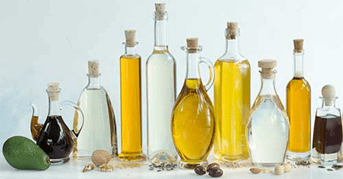Fig: Variety of oils

Q.20. Explain the following terms with one example each.
(a) Corrosion
(b) Rancidity

Ans.
(a) Corrosion: It is the process in which metals are slowly eaten up by the action of air moisture or chemicals. For example rusting is a form of corrosion in which iron is eaten up by the action of air and moisture and a reddish brown coating of iron oxide is formed as shown in the following chemical reaction.
2Fe + 3H2O → Fe2O3 + 3H2
(b) Rancidity: When the substance containing oils and fats are exposed to air they get oxidised and become rancid due to which their smell, taste and colour change. This process is known as rancidity. For example when a when butter is kept open for a long time then its smell and taste gets changed.

The document Chemical Reactions & Equations NCERT Solutions | Science & Technology for UPSC CSE is a part of the UPSC Course Science & Technology for UPSC CSE.
All you need of UPSC at this link: UPSC

## Science & Technology for UPSC CSE

165 videos|449 docs|327 tests

## FAQs on Chemical Reactions & Equations NCERT Solutions - Science & Technology for UPSC CSE

 1. What is a chemical equation?Ans. A chemical equation is a symbolic representation of a chemical reaction in the form of symbols and formulas, where the reactants are written on the left-hand side and the products on the right-hand side, separated by an arrow. The arrow denotes the direction of the reaction and indicates the conversion of the reactants into products.
 2. What are the types of chemical reactions?Ans. There are different types of chemical reactions, such as combination or synthesis reaction, decomposition reaction, displacement reaction, double displacement reaction, and redox reaction. Combination or synthesis reaction involves the combination of two or more reactants to form a single product. Decomposition reaction involves the breakdown of a single reactant into two or more products. Displacement reaction involves the exchange of atoms or ions between two reactants. Double displacement reaction involves the exchange of ions between two compounds. Redox reaction involves the transfer of electrons between reactants.
 3. What is the law of conservation of mass?Ans. The law of conservation of mass states that in a chemical reaction, the total mass of the reactants is equal to the total mass of the products. This law is based on the principle that matter cannot be created or destroyed, but it can be transformed from one form to another. Therefore, the number of atoms of each element present in the reactants must be equal to the number of atoms of the same element present in the products.
 4. What is the difference between exothermic and endothermic reactions?Ans. Exothermic reactions are those chemical reactions in which heat or energy is released to the surroundings. In contrast, endothermic reactions are those chemical reactions in which heat or energy is absorbed from the surroundings. In exothermic reactions, the products have lower energy than the reactants, whereas in endothermic reactions, the products have higher energy than the reactants.
 5. How can we balance a chemical equation?Ans. To balance a chemical equation, we need to ensure that the number of atoms of each element on the left-hand side of the equation is equal to the number of atoms of the same element on the right-hand side of the equation. We can balance the equation by adjusting the coefficients of the reactants and products. However, we cannot change the subscripts as they represent the number of atoms of each element in the molecule. The balanced chemical equation should satisfy the law of conservation of mass.

## Science & Technology for UPSC CSE

165 videos|449 docs|327 testsExplore Courses for UPSC exam### How to Prepare for UPSC

Read our guide to prepare for UPSC which is created by Toppers & the best Teachers
Signup to see your scores go up within 7 days! Learn & Practice with 1000+ FREE Notes, Videos & Tests.
10M+ students study on EduRev
Track your progress, build streaks, highlight & save important lessons and more!
Related Searches

,

,

,

,

,

,

,

,

,

,

,

,

,

,

,

,

,

,

,

,

,

;